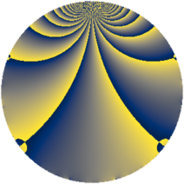# Properties

 Label 637.2.qLevel $637$ Weight $2$ Character orbit 637.q Rep. character $\chi_{637}(491,\cdot)$ Character field $\Q(\zeta_{6})$ Dimension $86$ Newform subspaces $10$ Sturm bound $130$ Trace bound $3$

# Related objects

## Defining parameters

 Level: $$N$$ $$=$$ $$637 = 7^{2} \cdot 13$$ Weight: $$k$$ $$=$$ $$2$$ Character orbit: $$[\chi]$$ $$=$$ 637.q (of order $$6$$ and degree $$2$$) Character conductor: $$\operatorname{cond}(\chi)$$ $$=$$ $$13$$ Character field: $$\Q(\zeta_{6})$$ Newform subspaces: $$10$$ Sturm bound: $$130$$ Trace bound: $$3$$ Distinguishing $$T_p$$: $$2$$, $$3$$

## Dimensions

The following table gives the dimensions of various subspaces of $$M_{2}(637, [\chi])$$.

Total New Old
Modular forms 148 106 42
Cusp forms 116 86 30
Eisenstein series 32 20 12

## Trace form

 $$86 q + 3 q^{2} + 2 q^{3} + 39 q^{4} + 12 q^{6} - 35 q^{9} + O(q^{10})$$ $$86 q + 3 q^{2} + 2 q^{3} + 39 q^{4} + 12 q^{6} - 35 q^{9} - 9 q^{10} - 6 q^{11} + 8 q^{12} + q^{13} - 36 q^{15} - 29 q^{16} + q^{17} + 6 q^{19} + 9 q^{20} - 12 q^{22} + 4 q^{23} - 6 q^{24} - 40 q^{25} + 39 q^{26} - 4 q^{27} - 11 q^{29} + 38 q^{30} - 27 q^{32} + 30 q^{33} + 31 q^{36} - 15 q^{37} - 16 q^{38} - 6 q^{39} - 98 q^{40} - 21 q^{41} - 16 q^{43} + 3 q^{45} - 6 q^{46} - 8 q^{48} + 78 q^{50} + 12 q^{51} - 4 q^{52} + 14 q^{53} - 24 q^{54} + 6 q^{55} - 93 q^{58} - 30 q^{59} - 13 q^{61} - 2 q^{62} + 38 q^{64} + 11 q^{65} + 52 q^{66} + 42 q^{67} + 11 q^{68} - 16 q^{69} - 24 q^{71} + 45 q^{72} - 21 q^{74} - 42 q^{75} + 24 q^{76} - 42 q^{78} + 96 q^{79} + 87 q^{80} - 23 q^{81} - 23 q^{82} + 27 q^{85} + 8 q^{87} + 38 q^{88} + 24 q^{89} - 30 q^{90} - 40 q^{92} + 60 q^{93} + 2 q^{94} + 56 q^{95} - 18 q^{97} + O(q^{100})$$

## Decomposition of $$S_{2}^{\mathrm{new}}(637, [\chi])$$ into newform subspaces

Label Dim $A$ Field CM Traces $q$-expansion
$a_{2}$ $a_{3}$ $a_{5}$ $a_{7}$
637.2.q.a $2$ $5.086$ $$\Q(\sqrt{-3})$$ None $$-3$$ $$2$$ $$0$$ $$0$$ $$q+(-1-\zeta_{6})q^{2}+(2-2\zeta_{6})q^{3}+\zeta_{6}q^{4}+\cdots$$
637.2.q.b $2$ $5.086$ $$\Q(\sqrt{-3})$$ None $$3$$ $$-1$$ $$0$$ $$0$$ $$q+(1+\zeta_{6})q^{2}+(-1+\zeta_{6})q^{3}+\zeta_{6}q^{4}+\cdots$$
637.2.q.c $2$ $5.086$ $$\Q(\sqrt{-3})$$ None $$3$$ $$1$$ $$0$$ $$0$$ $$q+(1+\zeta_{6})q^{2}+(1-\zeta_{6})q^{3}+\zeta_{6}q^{4}+\cdots$$
637.2.q.d $4$ $5.086$ $$\Q(\sqrt{-3}, \sqrt{-13})$$ None $$-6$$ $$0$$ $$0$$ $$0$$ $$q+(-2+\beta _{2})q^{2}+(1-\beta _{2})q^{4}+\beta _{3}q^{5}+\cdots$$
637.2.q.e $4$ $5.086$ $$\Q(\sqrt{-3}, \sqrt{-7})$$ None $$3$$ $$-1$$ $$0$$ $$0$$ $$q+(1-\beta _{3})q^{2}+(1-2\beta _{1}-2\beta _{2}+\beta _{3})q^{3}+\cdots$$
637.2.q.f $4$ $5.086$ $$\Q(\sqrt{-3}, \sqrt{-7})$$ None $$3$$ $$1$$ $$0$$ $$0$$ $$q+(1-\beta _{3})q^{2}+(-1+2\beta _{1}+2\beta _{2}-\beta _{3})q^{3}+\cdots$$
637.2.q.g $12$ $5.086$ 12.0.$$\cdots$$.1 None $$0$$ $$-3$$ $$0$$ $$0$$ $$q+\beta _{10}q^{2}+(\beta _{1}+\beta _{4}+\beta _{6})q^{3}+(\beta _{1}+\cdots)q^{4}+\cdots$$
637.2.q.h $12$ $5.086$ 12.0.$$\cdots$$.1 None $$0$$ $$0$$ $$0$$ $$0$$ $$q-\beta _{8}q^{2}+(-\beta _{4}-\beta _{9})q^{3}+(1-\beta _{2}+\cdots)q^{4}+\cdots$$
637.2.q.i $12$ $5.086$ 12.0.$$\cdots$$.1 None $$0$$ $$3$$ $$0$$ $$0$$ $$q+\beta _{10}q^{2}+(-\beta _{1}-\beta _{4}-\beta _{6})q^{3}+(\beta _{1}+\cdots)q^{4}+\cdots$$
637.2.q.j $32$ $5.086$ None $$0$$ $$0$$ $$0$$ $$0$$

## Decomposition of $$S_{2}^{\mathrm{old}}(637, [\chi])$$ into lower level spaces

$$S_{2}^{\mathrm{old}}(637, [\chi]) \cong$$ $$S_{2}^{\mathrm{new}}(13, [\chi])$$$$^{\oplus 3}$$$$\oplus$$$$S_{2}^{\mathrm{new}}(91, [\chi])$$$$^{\oplus 2}$$